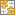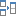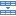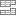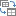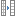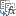# How to calculate days since or until date in Excel

Are you stuck with calculating how many days there are since a certain date or until date? This tutorial will teach you an easy way to add and subtract days from date in Excel. With our formulas you can quickly calculate 90 days from date, 45 days before date, and count whatever number of days you need.

Calculating days from date sounds like an easy task. However, this generic phrase can imply many different things. You may want to find a given number of days after date. Or you may wish to get the number of days from a certain date until today. Or you may be looking to count days from date to date. In this tutorial, you will find solutions to all these and a lot more tasks.

## Days from/before date calculator

Want to find a date that occurs 60 days from a specific date or determine 90 days before date? Supply your date and the number of days in the corresponding cells, and you will get the results in a moment:

## How many days since / until date calculator

With this calculator, you can find how many days are left to a certain date, for example your birthday, or how many days have passed since your birthday:

Tip. To find out how many days there are from date to date, use the Days Between Dates Calculator.

## How to calculate days from date in Excel

To find a date that is N days from a certain date, just add the required number of days to your date:

Date + N days

The key point is to supply the date in the format that Excel understands. I'd suggest using the default date format or convert a text-date to a serial number representing the date with DATEVALUE or explicitly specify year, month and day with the DATE function.

For example, here's how you can add days to April 1, 2018:

90 days from date
`="4/1/2018"+90`

60 days from date
`="1-Apr-2018"+60`

45 days from date
`=DATEVALUE("1-Apr-2018")+45`

30 days from date
`=DATE(2018,4,1)+30`

To get a more universal days from date formula, enter both values (source date and the numbers of days) in separate cells and reference those cells. With the target date in B3 and the number of days in B4, the formula is as simple as adding up two cells:

`=B3+B4`

As plain as it could possibly be, our formula works just perfectly in Excel: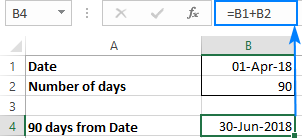With this approach, you can easily calculate the expiry or dues dates for a whole column. As an example, let's find 180 days from date.

Supposing you have a list of subscriptions that expire in 180 days after the purchase date . With the order date in B2, you enter the following formula in, say C2, and then copy the formula to the entire column by double-clicking the fill handle:

`=B2+180`

The relative reference (B2) forces the formula to change based on a relative position of each row: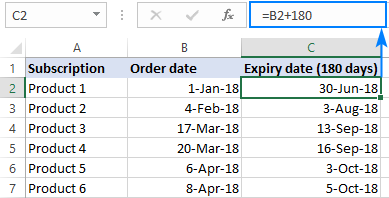You can even calculate a few intermediate dates for each subscription, all with a single formula! For this, insert a couple of new columns and indicate when each of the dates is due (please see the screenshot below):

• 1st reminder: 90 days from purchase date (C2)
• 2nd reminder: 120 days from purchase date (D2)
• Expiration: 180 days from purchase date (E2)

Write the formula for the first cell that calculates the 1st reminder date based on the order date in B3 and the number of days in C2:

`=\$B3+C\$2`

Please notice that we fix the column coordinate of the first reference and the row coordinate of the second refence with the \$ sign so that the formula copies correctly to all other cells. Now, drag the formula rightwards and downwards until the last cells with data, and make sure it calculates the due dates in each column appropriately (please notice that the second reference changes for each column while the first reference is locked to column B):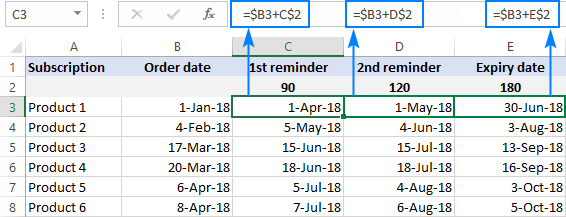Note. If the results of your calculations are displayed as numbers, apply the Date format to the formula cells to have them displayed as dates.

## How to calculate days before date in Excel

To find a date that is N days before a certain date, perform the arithmetic operation of subtraction instead of addition:

Date - N days

As with adding days, it is important that you enter the date in the format understandable to Excel. For example, that's how you can subtract days from a given date, say from April 1, 2018:

90 days before date
`="4/1/2018"-90`

60 days before date
`="1-Apr-2018"-60`

45 days before date
`=DATE(2018,4,1)-45`

Naturally, you can enter both values in individual cells, say date in B1 and the number of days in B2, and subtract the "days" cell from the "date" cell:
`=B1-B2`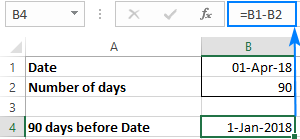## How to count days until date

To calculate the number of days before a certain date, subtract today's date from that date. And to supply the current date that updates automatically, you use the TODAY function:

Date - TODAY()

For example, to find how many days are left until January 31, 2018, use this formula:
`="12/31/2018"-TODAY()`

Or, you can enter the date in some cell (B2) and subtract today's date from that cell:
`=B2-TODAY()`

In a similar manner, you can find difference between two dates, simply by subtracting one date from another.

You can even concatenate the returned number with some text to create a nice-looking countdown in your Excel. For example:
`="Just "& A4-TODAY() &" days left until Christmas!"`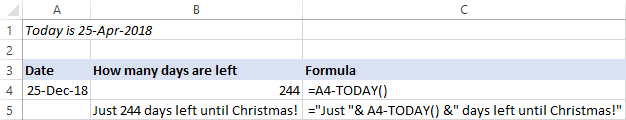Note. If your count days formula shows a date, set the General format to the cell to display the result as a number.

## How to count days since date

To calculate how many days have passed since a certain date, you do the opposite: subtract the date from today:

TODAY() - Date

As an example, let's find the number of days since your last birthday. For this, enter your date in A4, and subtract the current date from it:
`=A4-TODAY()`

Optionally, add some text explaining what that number is:
`=TODAY()-A4 &" days since my birthday"`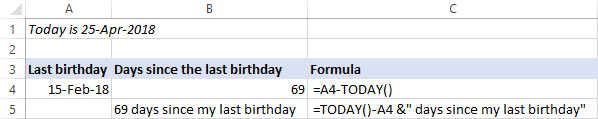## How to calculate working days from date

Microsoft Excel provides 4 different functions to calculate weekdays. The detailed explanation of each function can be found here: How to calculate weekdays in Excel. For now, let's just focus on practical uses.

### Calculate N business days from/before date

To return a date that is a given number of working days ahead of or prior to the start date that you specify, use the WORKDAY function.

Here are a couple of formula examples to get a date that occurs exactly N business days from a certain date:

30 business days from April 1, 2018
`=WORKDAY("1-Apr-2018", 30)`

100 working days from the date in A1:
`=WORKDAY(A1, 100)`

To find a date that occurred a specified number of business days before a given date, supply the days as a negative number (with the minus sign). For example:

120 business days before April 1, 2018
`=WORKDAY("1-Apr-2018", -120)`

90 working days before the date in A1:
`=WORKDAY(A1, -90)`

Or, you can enter both values in predefined cells, say B1 and B2, and your business days calculator can look something similar to this:

Workdays from a given date:
`=WORKDAY(B1, B2)`

Workdays before a given date:
`=WORKDAY(B1, -B2)`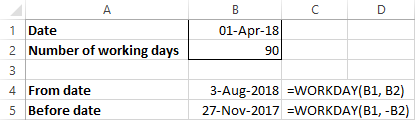Tip. The WORKDAY function calculates days based on the standard working calendar, with Saturday and Sunday as weekend days. If your working calendar is different, then use the WORKDAY.INTL function that allows specifying custom weekend days.

### Count business days since/until date

To return the number of days between two dates excluding Saturdays and Sundays, use the NETWORKDAYS function.

To find out how many working days are left until a certain date, supply the TODAY() function in the first argument (start_date) and your date in the second argument (end_date).

For example, to get the number of days until the date in A4, use this formula:
`=NETWORKDAYS(TODAY(), A4)`

Of course, you are free to concatenate the returned count with your own message like we did in the above examples.

For instance, let's see how many business days are left until the end of 2018. For this, enter 31-Dec-2018 in A4 as a date, not text, and use the following formula to get the number of working days until this date:

`="Only "&NETWORKDAYS(TODAY(), A4)&" work days until the end of the year!"`

Wow, only 179 working days are left! Not as many as I thought :)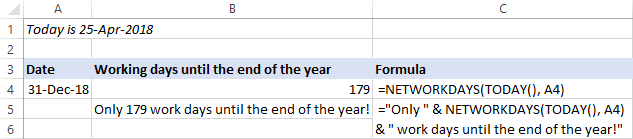To get the number of business days since a given date, reverse the order of arguments - enter your date in the first argument as the start date and TODAY() in the second argument as the end date:
`=NETWORKDAYS(A4, TODAY())`

Optionally, display some explanatory text like this:
`=NETWORKDAYS(A4, TODAY())&" work days since the beginning of the year"`

Only 83 working days… I thought I had worked at least 100 days this year already!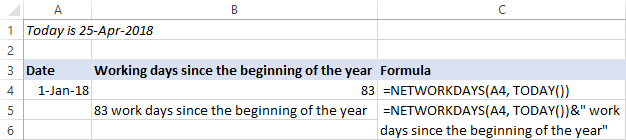Tip. To specify your own weekends other than Saturday and Sunday, use the NETWORKDAYS.INTL function.

## Date and Time Wizard - quick way to calculate days in Excel

This wizard is kind of Swiss army knife for Excel date calculations, it can compute almost anything! You just select the cell where you want to output the result, click the Date & Time Wizard button on the Ablebits Tools tab and specify how many days, weeks, months or years (or any combination of these units) you want to add to or subtract from the source date.

As an example, let's find out what date is 120 days< from date in B2: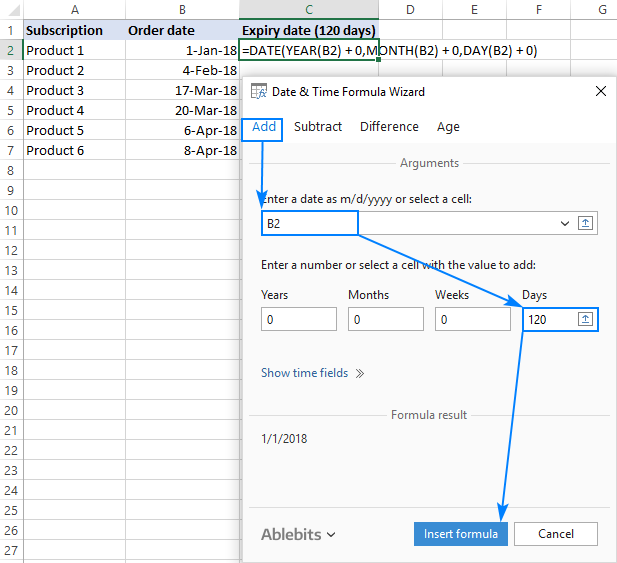Click the Insert formula button to enter the formula in the selected cell, and then copy it to as many cells as you need: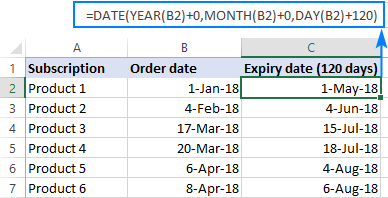As you may have noticed, the formula built by the wizard is different from the ones we've used in the previous examples. It's because the wizard is designed to calculate all possible units, not only days.

To get a date that occurred N days before a certain date, switch to the Subtract tab, input the source date in the corresponding box, and specify how many days you want to subtract from it. Or, enter both values in separate cells, and get a more flexible formula that recalculates with every change you make to the original data: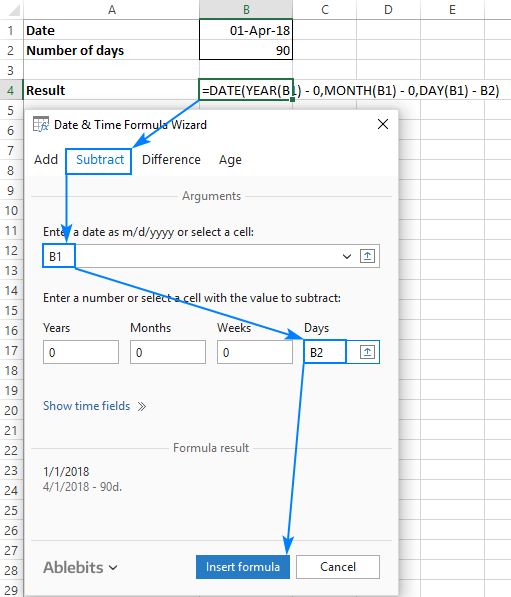## Date Picker - calculate days in drop-down calendar

There exist a great number of third-party drop-down calendars for Excel, both free and paid. All of them can insert a date into a cell with a click. But how many Excel calendars can also calculate dates? Our Date Picker can!

You simply select a date in the calendar and click the Date Calculator icon or press the F4 key: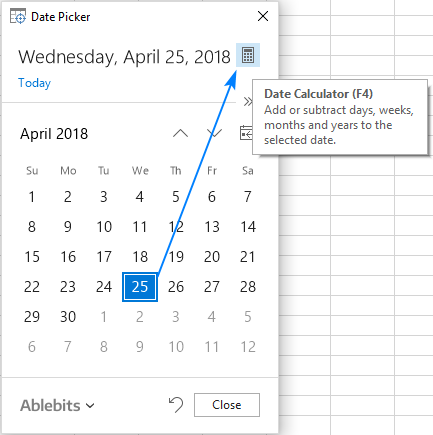Then, click the Day unit on the preview pane and type the number of days to add or subtract (you choose which operation to perform by clicking the plus or minus sign on the input pane).

Finally, press the Enter key to insert the calculated date into the currently selected cell or press F6 to display the date in the calendar. Alternatively, click one of the buttons shown in the image below. In this example, we are calculating a date that is 60 days from April 1, 2018: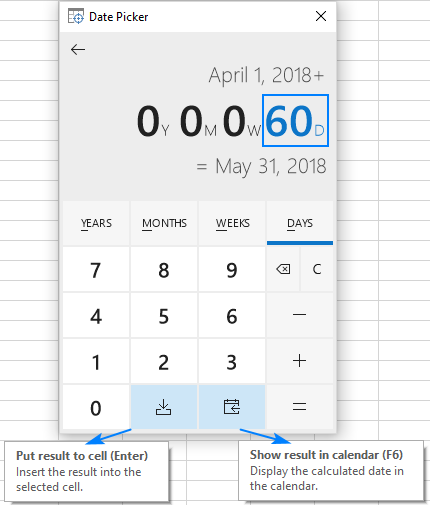That's how you find days from or before a certain date in Excel. I have a closer look the formulas discussed in this tutorial, you are welcome to download our sample workbook to Calculate Days from Date. I thank you for reading and hope to see you on our blog next week!

### 15 responses to "How to calculate days since or until date in Excel"

1.PolkaDotsEmpire says:

Svetlana Cheusheva, thank you for this post. Its very inspiring.

2.roasous says:

1982-2014 CSRC.2015.007

3.IvTe says:

I have a monthly report but need to count only daily data. I don't know to use Today option if formula is =COUNTIF(A1:A50,"Vacation")=TODAY(). Please help

•Doug says:

IvTe:
If you can use COUNTIFS in your version of Excel then I believe this formula is what you're looking for.
=COUNTIFS(A1:A50,"=VACA*",B1:B50,"<="&TODAY())
Where the word "VACATION" is in A1:A50 and the Date is in B1:B50. Note this formula uses a wildcard so "Vaca" is counted, too.

4.Brenda says:

What is the date four days before November first 2018 without counting the weekend

•Svetlana Cheusheva (Ablebits.com Team) says:

Hi Brenda,

The below formula says it's October 26, 2018:

=WORKDAY("1-Nov-2018", -4)

Please remember to apply the Date format to the formula cell for the result to display correctly.

•WILSON says:

I NEED A EXCEL FORMULA TO calculate 15 days free storage

5.Suresh says:

HI,
Would like to calculate the due days based on the present days.

6.Nikhil Srinivasan says:

Thanks a lot

7.Eric Vo says:

Bonjour,

J'aimerais trouver une formule pour calculer le nombre de jours depuis une date jusqu'aujourd'hui, SVP.
Je n'ai que la date entrée donc les formules de DATEDIF et DATEVAL ne peuvent pas marcher.
Ex: j'ai un dossier de 2017 qui n'est pas fini, que dois-je faire pour avoir le nombre de jours à partir de 2017 jusqu'aujourd'hui?

8.kelly says:

Hi: is there a modification to use to exclude holidays when calculating workdays using this formula:
=workday(A1, -2)

9.Harry says:

30 days late on payment and I want to make it update each date they are late.

Ex: 30days late making the next day(s) go up each day automatically So I don't have to do it myself each day

•Alexander Trifuntov (Ablebits.com Team) says:

Hello Harry!
Please describe your problem in more detail. Write an example of the source data and the result you want to get. It’ll help me understand it better and find a solution for you. Thank you.

10.Aoife Connolly says:

Hello,

I need to calculate days till something is due and then continuing counting how many days overdue (e.g. -3 days late). I have a formatted the due date and then in the column overdue what formula do i put?

Ultimate Suite for Microsoft Excel